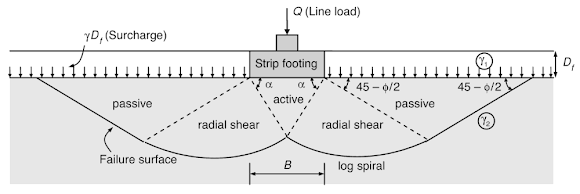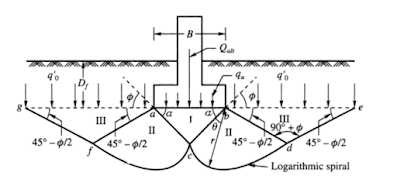# Terzaghi's Equation: Soil Bearing Capacity for Foundations

Terzaghi (1943) developed a rational bearing capacity equation for strip footing, by assuming the bearing capacity failure of the foundation in general shear mode. The theory was an extension of Prandtl's theory (1921).Fig.1. General Shear Failure Mode for Strip Footing - Terzaghi's Theory Assumed Bearing Capacity Failure

The assumptions and failure surface assumed for deriving the bearing capacity equation are explained in this article.

### Assumptions in Terzaghi's Bearing Capacity Equation

1. The soil is semi-infinite, homogeneous, and isotropic.
2. The problem is studied in two-dimensional.
3. The base of the footing is rough.
4. The ground surface is horizontal.
5. The failure is studied as a general shear failure.
6. The load acting on the footing is vertical and symmetrical.
7. The overburden pressure at the foundation level is equivalent to the surcharge load calculated as           qo = γDf, Where γ is the effective unit weight of the soil, and Df is the depth of the foundation less than the width of the foundation.
8. The principle of superposition can be applied.
9. Coulomb law, i.e. σ = C +  σ tan ф; is employed.

### Terzaghi's Bearing Capacity Equation

The Terzaghi's bearing capacity equation is given by:

qu = CNc + γ1DfNq + 0.5Bγ2Nγ

In the above equation, and as per Fig.1,

• qu= Ultimate Bearing Capacity of the soil
• C= Cohesion
• γ12= Unit weight of the soil above and below the footing level ( Fig.1)
• Nc,NqNγ= Bearing capacity factors that are a function of friction angle
• Df= Depth of the foundation below the ground level (Fig.1)
In the above equation:

• CNc = Contribution of cohesion
• γ1DfNq = Frictional contribution of overburden pressure or surcharge
• 0.5Bγ2Nγ = Frictional contribution of self-weight of soil in the failure zone
The bearing capacity factors Nc,Nq,Nγ are determined by the following equations:

### Terzaghi's Bearing Capacity Theory

Terzaghi's bearing capacity equation was derived by assuming a general shear failure of soil under the action of an ultimate load per unit area (qu) as shown in Fig.2. Under the action of this load, failure surfaces are formed below the footing, which divides the soil into three zones of plastic equilibrium. The three zones are:
1. Zone 1 : Elastic Equilibrium
2. Zone II :  Radial Shear
3. Zone III: Rankine Passive StateFig.2. General Shear Failure Surface as Assumed by Terzaghi for a Strip Footing

The sinking of Zone 1 creates two zones of plastic equilibrium, i.e. Zone II and Zone III, on either side of the footing as shown in Fig.2. Zone II is the radial shear zone whose remote boundaries bd and af meet the horizontal surface at angles ( 45 - Φ/2), whereas Zone III is a passive Rankine zone.

The boundaries de and fg of these zones are straight lines and they meet the surfaces at angles ( 45 - Φ/2). The curved parts cd and cf in Zone II are parts of logarithmic spirals whose centers are located at b and a respectively. Given Φ is the angle of shearing resistance.